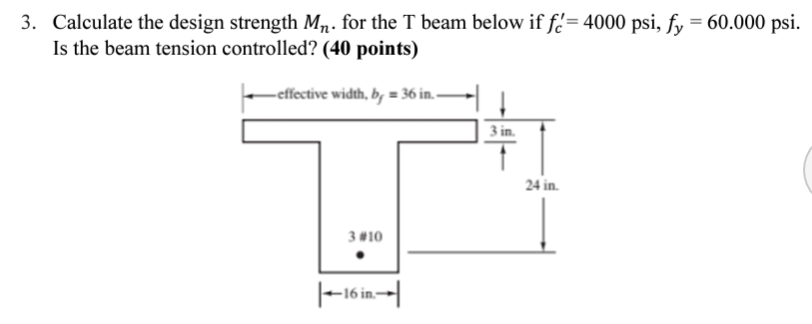Home / Expert Answers / Civil Engineering / 3-calculate-the-design-strength-mn-for-the-t-beam-below-if-fc-4000psi-fy-60-000ps-pa153

# (Solved): 3. Calculate the design strength Mn. for the T beam below if fc=4000psi,fy=60.000ps ...3. Calculate the design strength . for the T beam below if . Is the beam tension controlled? (40 points)

We have an Answer from Expert